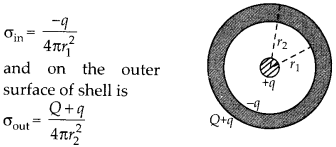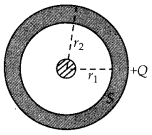Enlightened

# Question 15: NCERT Solutions for 12th Class Physics: Chapter 2-Electrostatic Potential and Capacitance

• 0

Question 15: NCERT Solutions for 12th Class Physics: Chapter 2-Electrostatic Potential and Capacitance

A spherical conducting shell of inner radius r, and outer radius r2 has a charge Q.

1. A charge q is placed at the centre of the shell. What is the surface charge density on the inner and outer surfaces of the shell?
2. Is the electric field inside a cavity (with no charge) zero, even if the shell is not spherical, but has any irregular shape? Explain.
Share

1. Solution:

1. Surface charge density on the inner surface of shell is1. The electric flux linked with any closed surface S inside the conductor is zero as electric field inside conductor is zero.
So, by Gauss’s theorem net charge enclosed by closed surface S is also zero i.e.,$q_{ net }=0$
So, if there is no charge inside the cavity, then there cannot be any charge on the inner surface of the shell and hence electric field inside the cavity will be zero, even though the shell may not be spherical.Check the complete chapter with solutions.

NCERT Solutions for 12th Class Physics: Chapter 2-Electrostatic Potential and Capacitance

• 0